Example Questions

1 2 8 9 10 11 12 13 14 16 Next →

Example Question #1062 : Act Math

You have an empty cone and a cylinder filled with water. The cone has a diameter ofand a height of. The cylinder has a diameter ofand a height of. If you dump the water from the cylinder into the cone until it is filled, what volume of water will remain in the cylinder?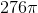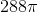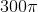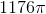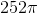Explanation:

1. Find the volumes of the cone and cylinder:

Cone:

Since the diameter is, the radius is.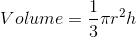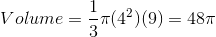Cylinder:

Since the diameter is, the radius is.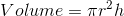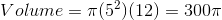2. Subtract the cone's volume from the cylinder's volume: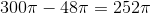Example Question #1063 : Act Math

What is the volume of a cone with a height of 7 cm and a radius of 4 cm? Leave your answer in terms ofand as a fraction if need be.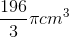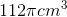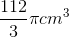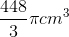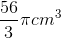Explanation:

To find the volume of a cone plug the radius and height into the formula for the volume of a cone.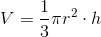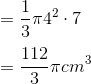Example Question #1064 : Act Math

Which of the following will quadruple the volume of a cone?

2. Doubling the height

1, 2 and 3

1 and 3

1 only

2 only

3 only

1 and 3

Explanation:

Bearing in mind the volume formula for a cone: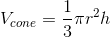Because the volume varies by the square of the radius, doubling the radius will quadruple the volume (since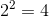.) Because the volume also varies linearly by the height, quadrupling the height will quadruple the volume.

Example Question #1065 : Act Math

What is the volume of a cone with a radius ofand a height of? Leave your answer in terms of, reduce all fractions.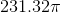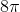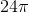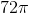Explanation:

To find the volume of a cone with radius, and heightuse the formula: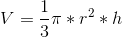.

We plug in our given radius and height to find: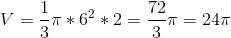Example Question #1066 : Act Math

A conical paper cup is being used as a makeshift funnel for motor oil. If the cup is 100 millimeters deep at the center and has a radius of 70 millimeters, how many cubic millimeters of motor oil can it hold at one time? Round your final answer to the nearest integer.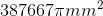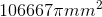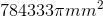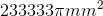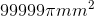Explanation:

The formula for the volume of a cone is: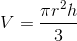With the information we have, we can simply plug values into this equation and solve.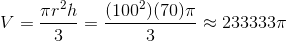So our cone can hold approximately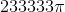cubic millimeters of motor oil.

Example Question #1067 : Act Math

Find the volume of a cone whose diameter isand height is.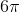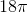Explanation:

To find the volume of a cone, simply use the following formula. Thus,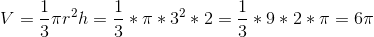Example Question #1068 : Act Math

Find the volume of a cone with radiusand height of.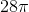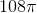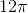Explanation:

To solve simply use the formula for the voluma of a cone. Thus,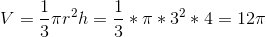Example Question #1069 : Act Math

What is the volume of a cone with a radius of 3 mm and a height of 6 mm?Explanation:

The formula for the volume of a cone is given by the equation: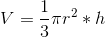.

Pluggin in our values we get: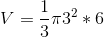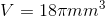1 2 8 9 10 11 12 13 14 16 Next →